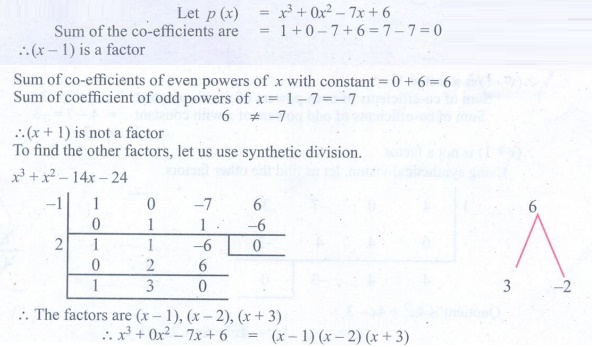Home | | Maths 9th std | Exercise 3.8: Factorisation using Synthetic Division

# Exercise 3.8: Factorisation using Synthetic Division

Maths : Algebra : Book Back, Exercise, Example Numerical Question with Answers, Solution : Exercise 3.8: Factorisation using Synthetic Division

Factorisation using Synthetic Division

Exercise 3.8

1. Factorise each of the following polynomials using synthetic division:

(i) x 3 3x2 10x + 24(ii) 2x3 3x2 3x + 2(iii) 7x + 3 + 4x3(iv) x3 + x2 14x 24(v) x 3 7 x + 6(vi) x 3 10x 2 x +10Tags : Numerical Problems with Answers, Solution | Algebra | Maths , 9th Maths : UNIT 3 : Algebra
Study Material, Lecturing Notes, Assignment, Reference, Wiki description explanation, brief detail
9th Maths : UNIT 3 : Algebra : Exercise 3.8: Factorisation using Synthetic Division | Numerical Problems with Answers, Solution | Algebra | Maths